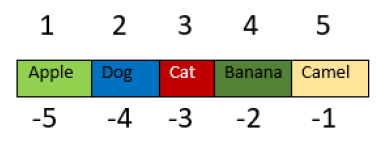# How to create an array in PowerShell?

To create or declare an array in PowerShell, there are few methods.

You can directly assign values to the variable and separate it by comma (,) and that variable becomes the variable.

For example,

$a = "Apple","Dog","Cat","Banana","Camel"$a is now an array. To access the variable you can use the Indexing method. The first value will be stored at 0, second at 1 and so on.

$a will give the output “Apple”,$a will give the output “Dog” and so on. Negative indexing also works in the array. -1 is the last index, -2 is the second last and so on. $a[-1] will give the output “Camel”. For better understanding see the picture demonstration below.You can create a sequential array with the shortcut below. $a = 1..5

When you check the output, the array will be created.

PS C:\WINDOWS\system32> $a 1 2 3 4 5 You can also create an array with different data types, such arrays are called the Polymorphic arrays. For example, $a = "Hello",'c',10,34.3

Here we have created an array with the values. You can also create an empty array. This is generally used when working with scripts. Arrays are created initially and then values are assigned.

To create a null array,

$a = @() When you check the length of the array you will get 0 size. $a.Length

PS C:\WINDOWS\system32>$a.Length You can assign values to the array as below, $a = @(1,"Hi",45.6)